Download the eSaral app and start learning from Kota's top IITians and doctors.

# NCERT Solutions For Class 11 Maths Chapter 8 Miscellaneous Exercise - Binomial Theorem - Free PDF Download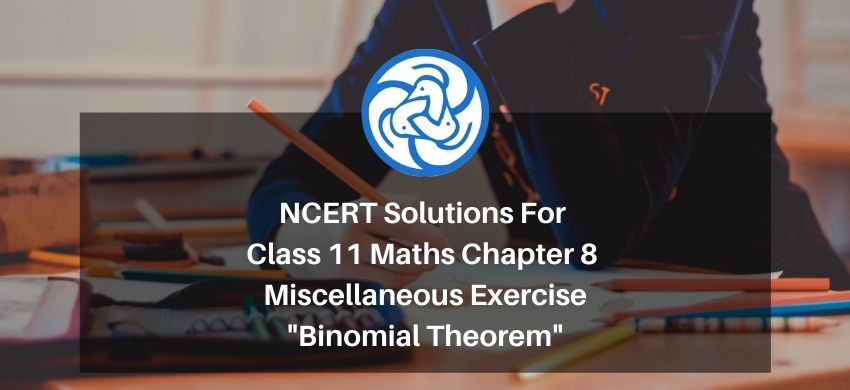Hey, are you a class 11 Student and Looking for Ways to Download NCERT Solutions For Class 11 Maths Chapter 8 Miscellaneous Exercise? If Yes then you are at the right place.

All the solutions of Class 11 Maths Chapter 8 Miscellaneous Exercise in PDF is prepared by Kota’s top IITian’s Faculties by keeping Simplicity in mind.

If you want to score high in your class 11 Maths Exam then it is very important for you to have a good knowledge of all the important topics, so to learn and practice those topics you can use eSaral NCERT Solutions.

So, without wasting more time Let’s start.

### Download The PDF of NCERT Solutions For Class 11 Math Chapter 8 Miscellaneous Exercise "Binomial Theorem"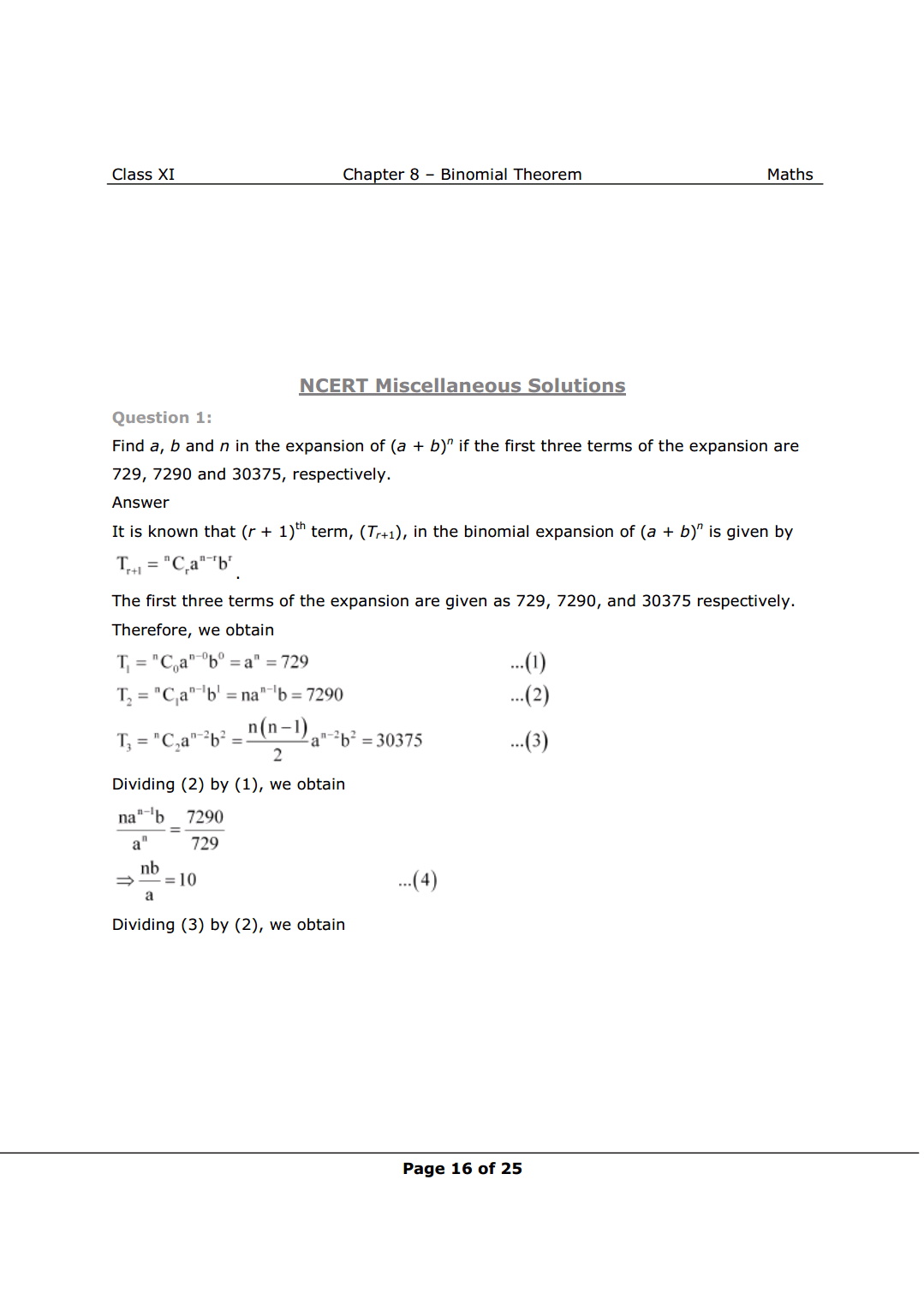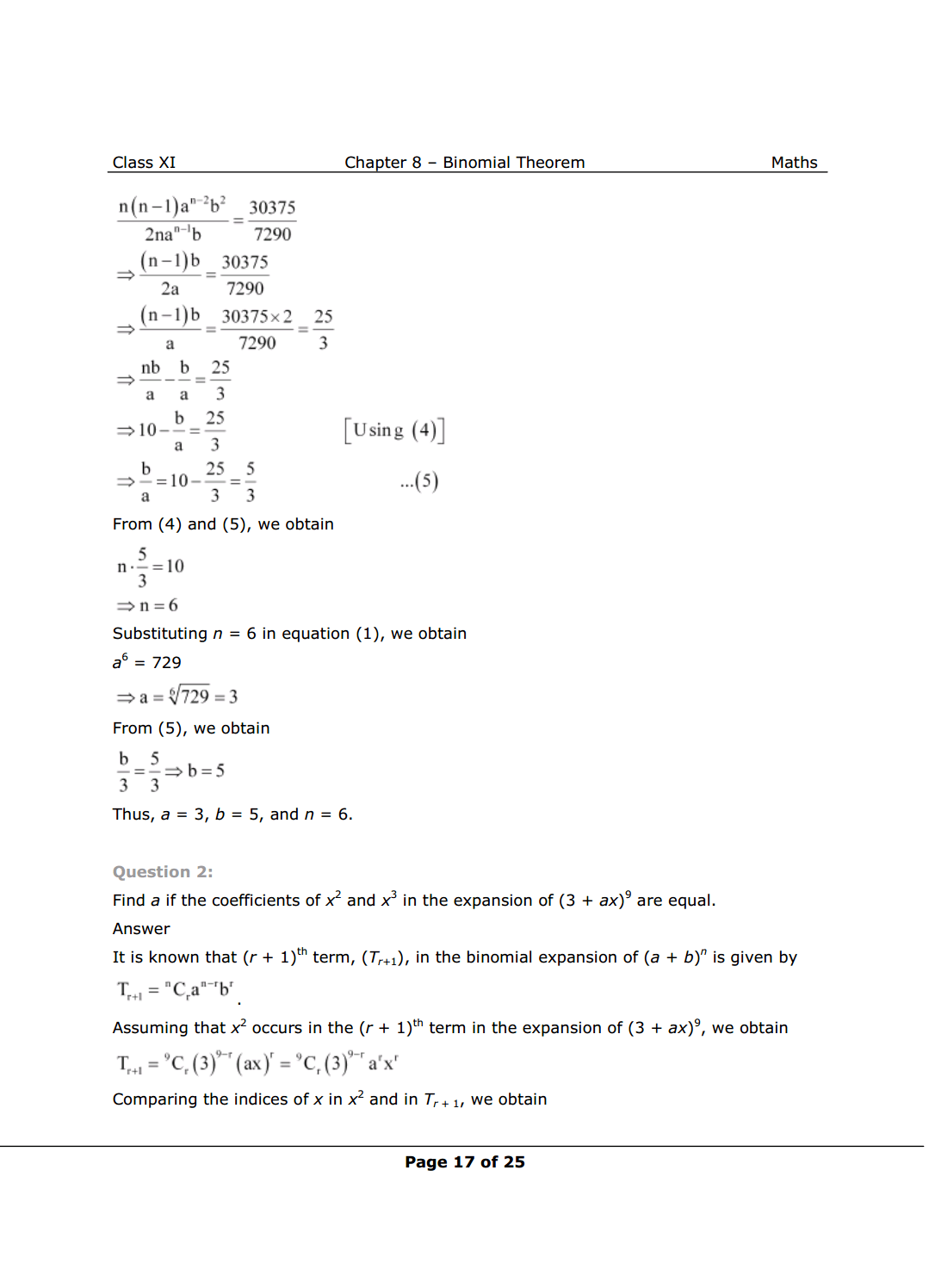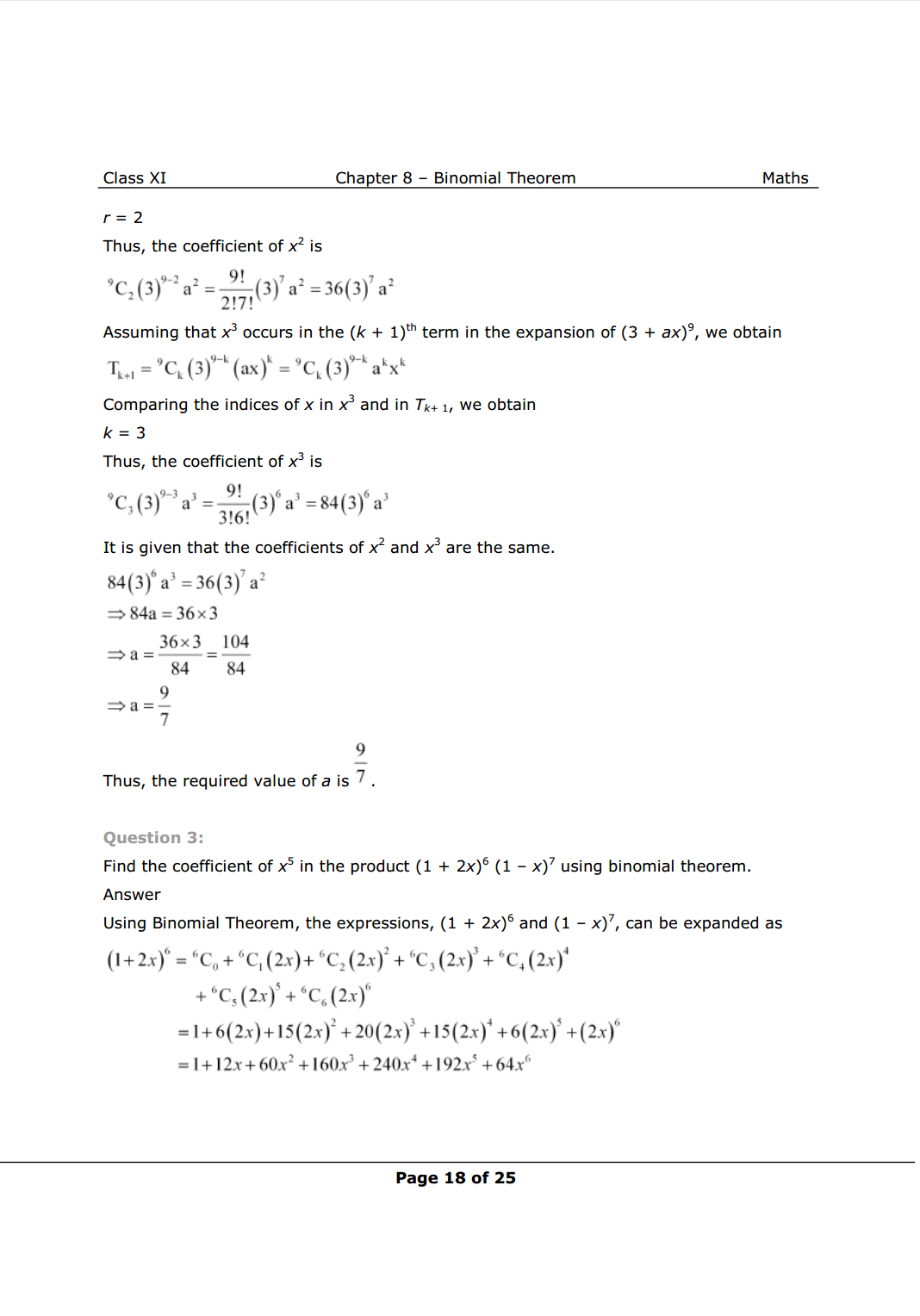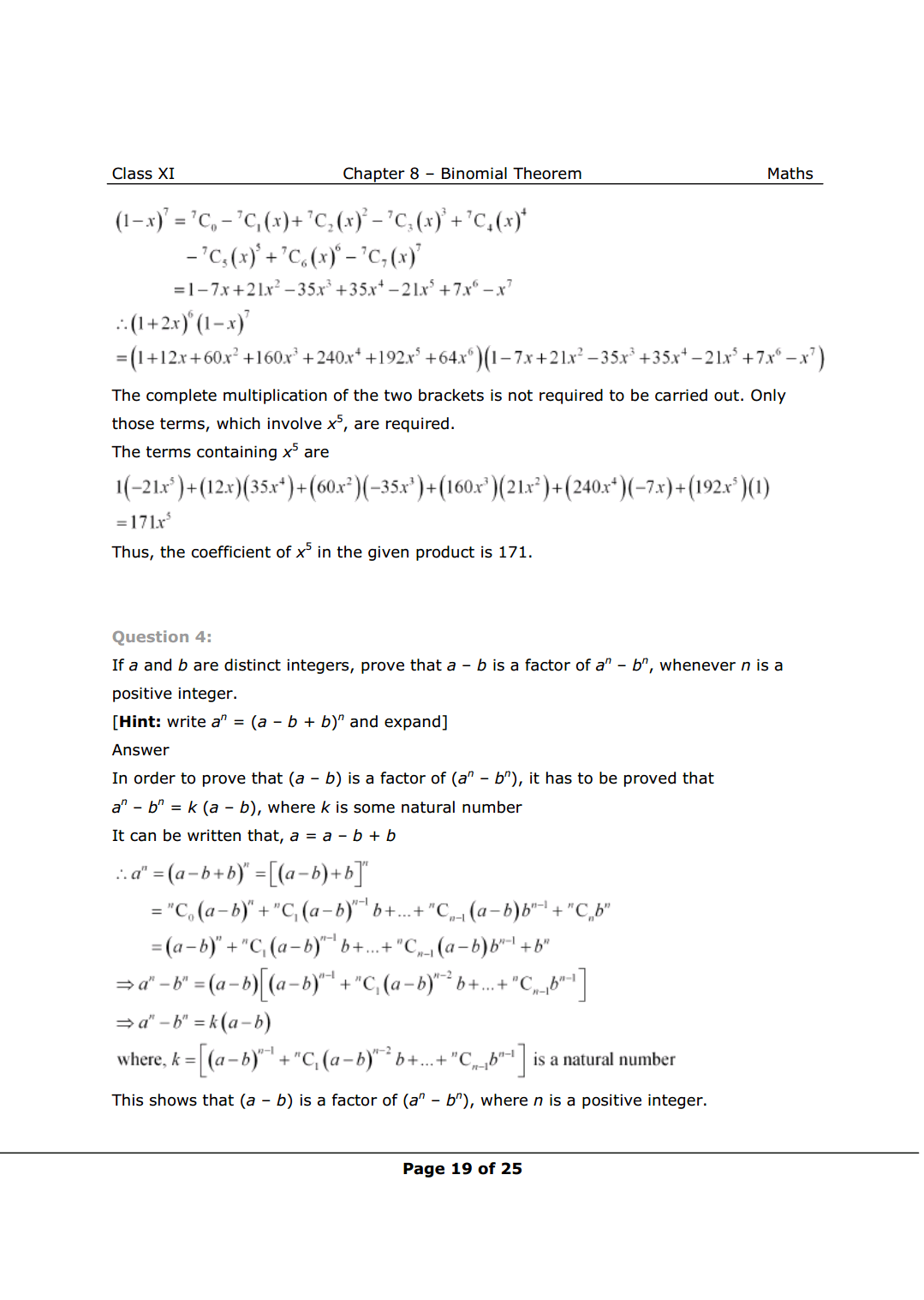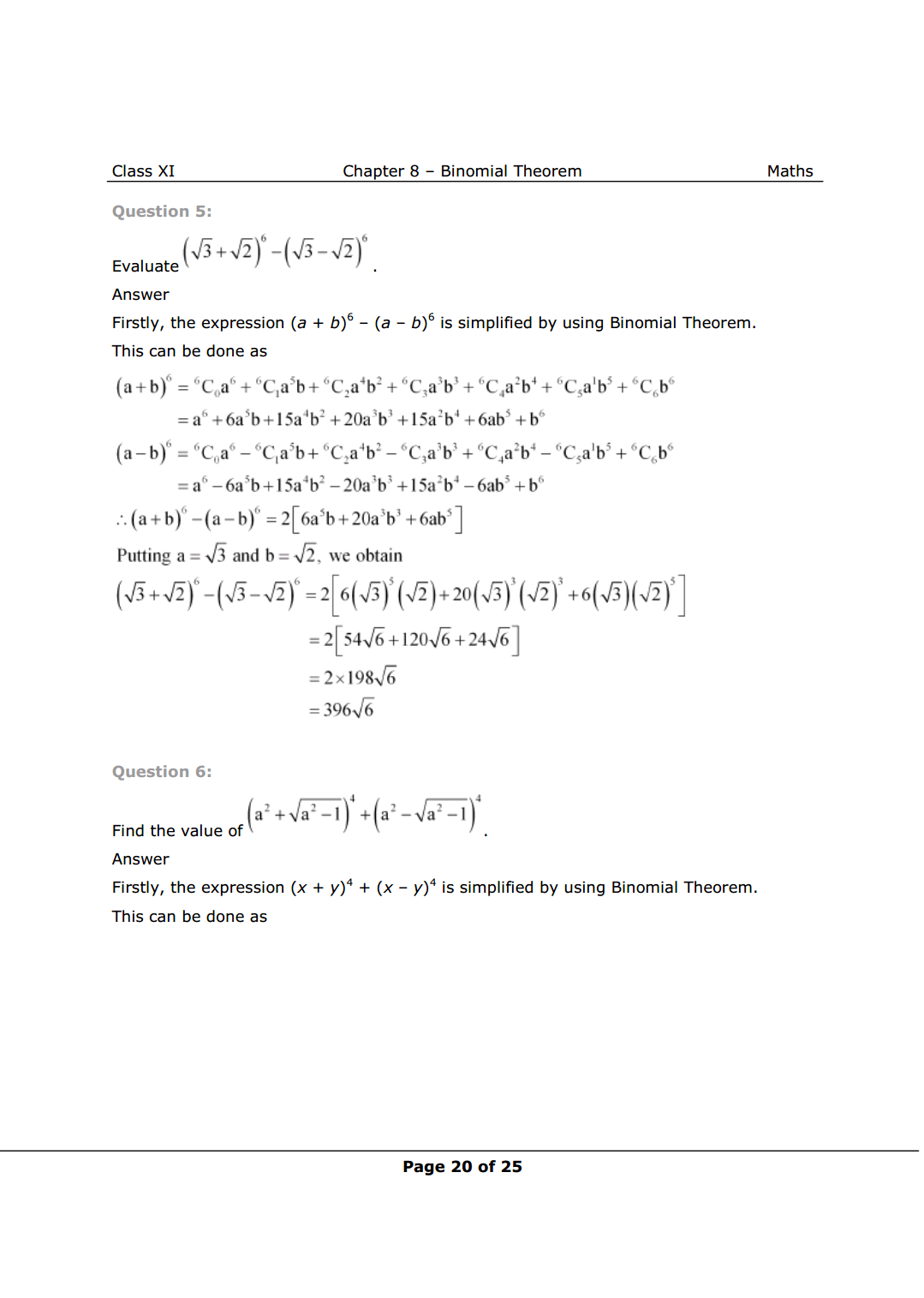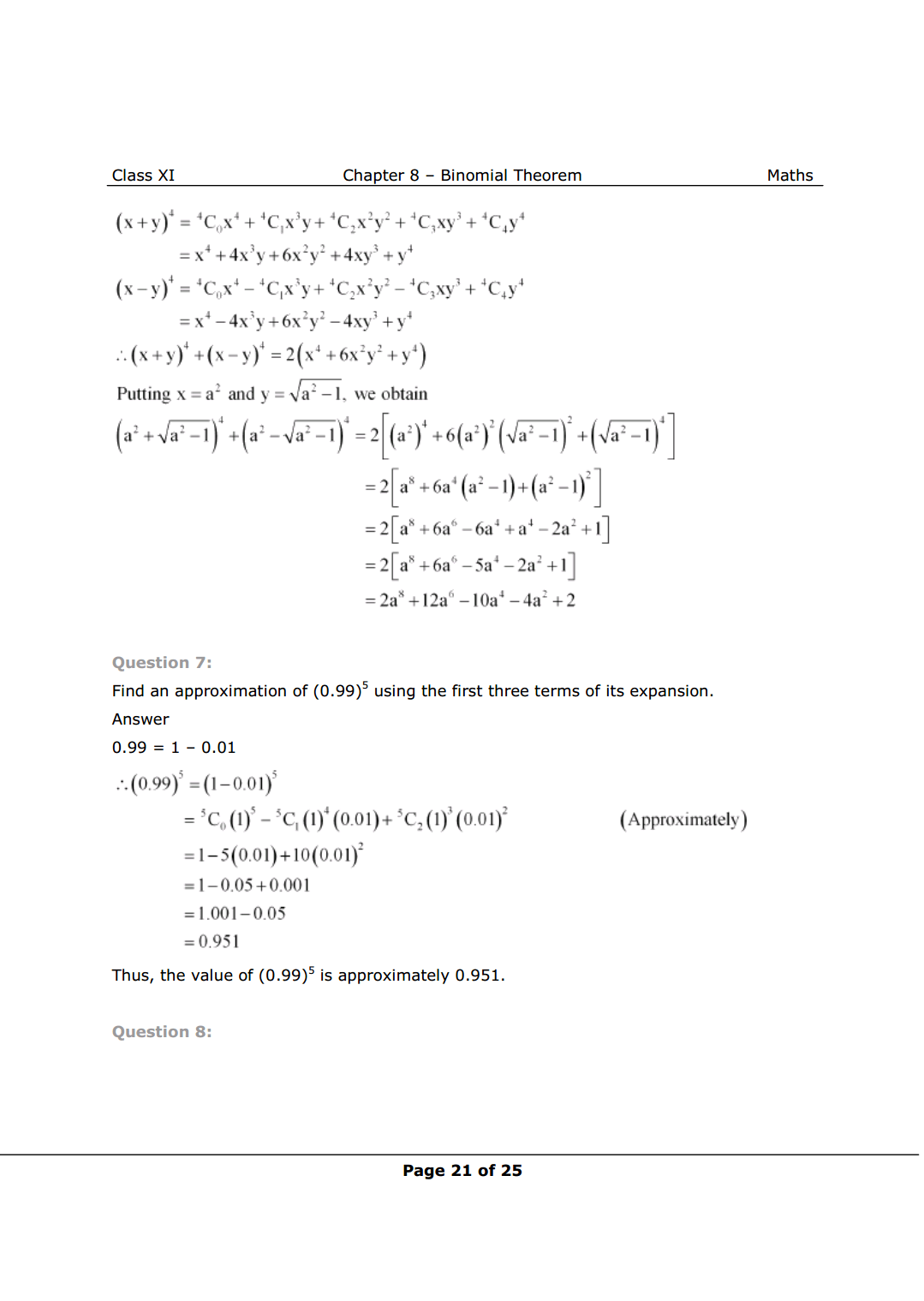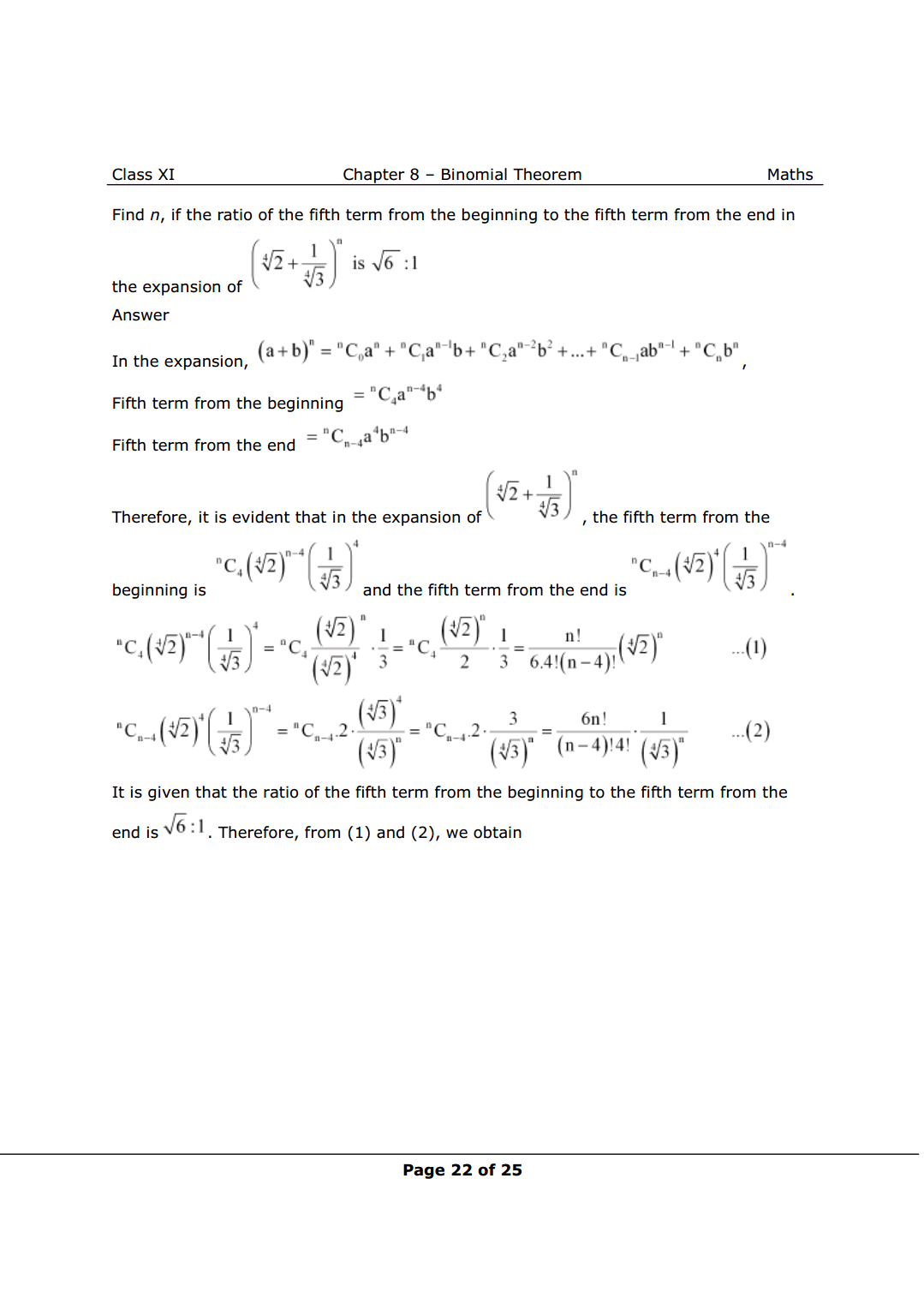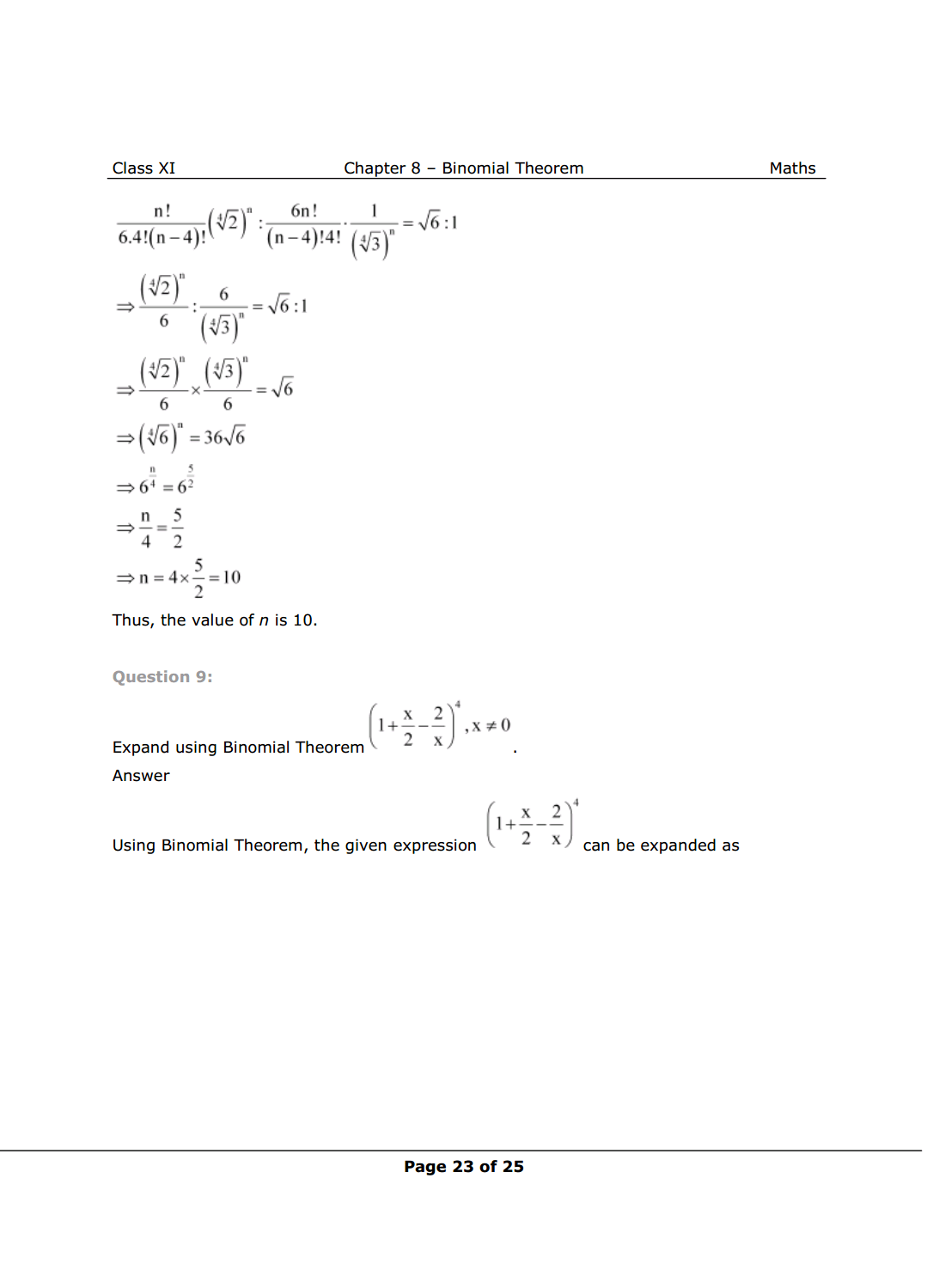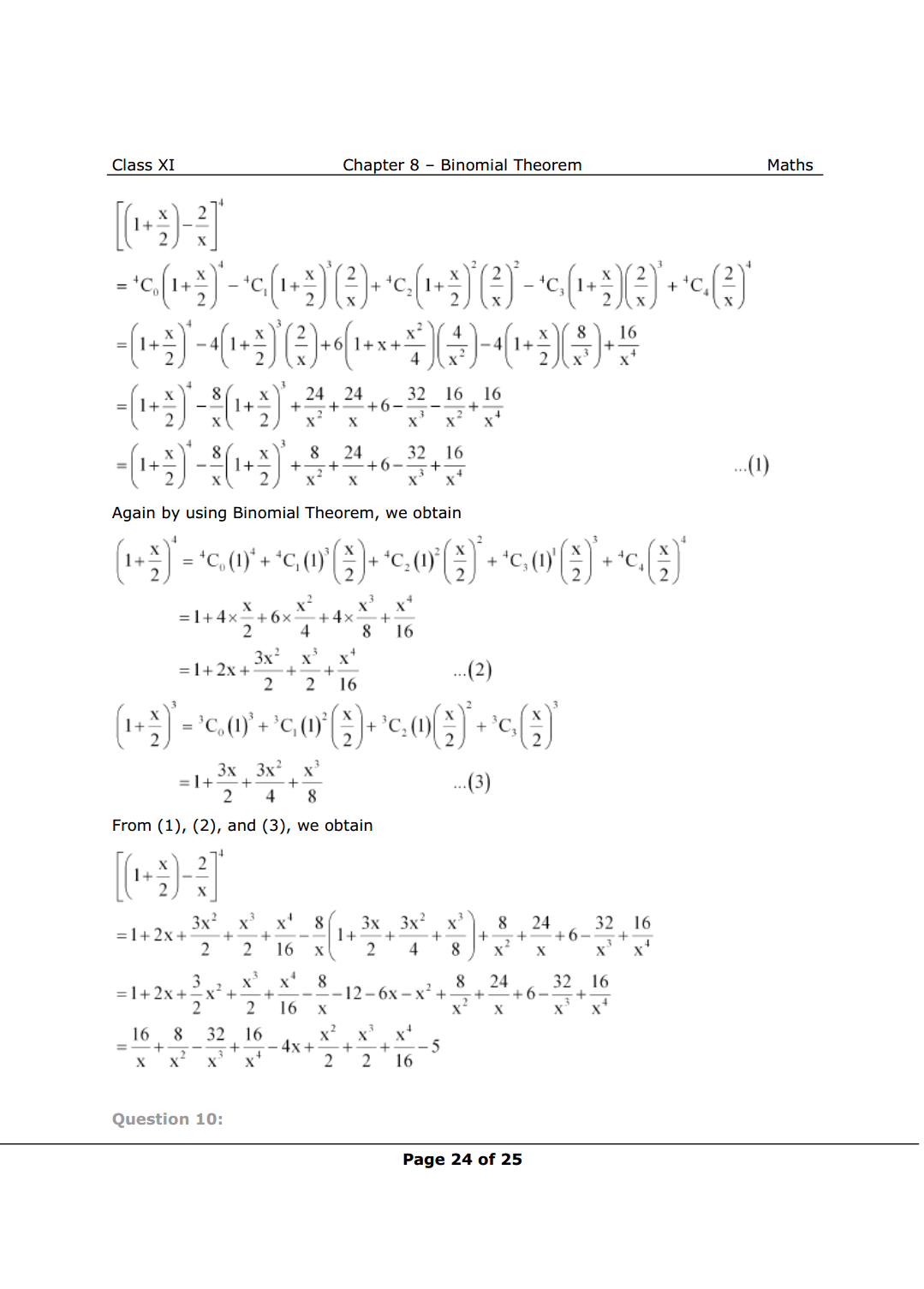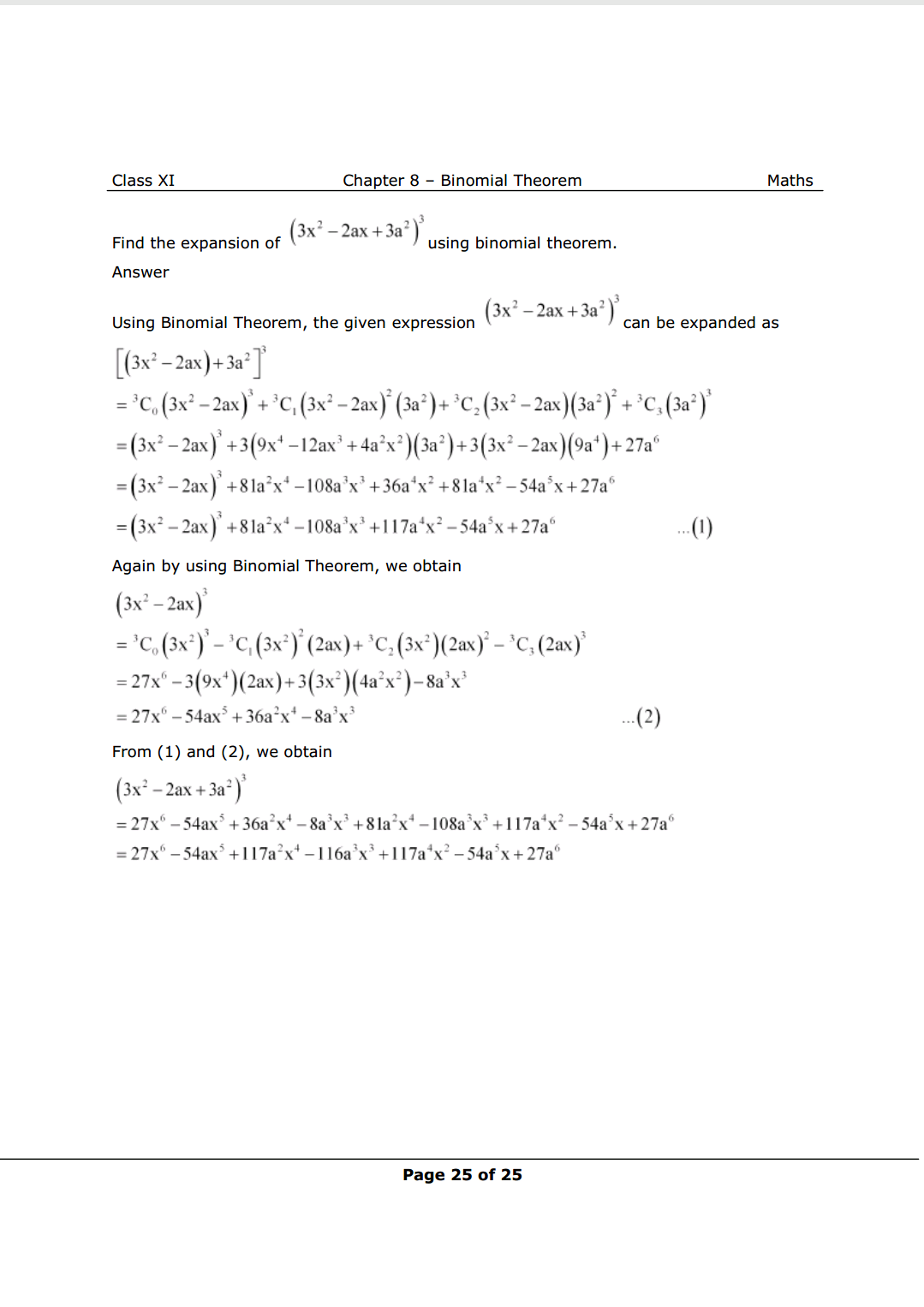NCERT Class 11 Math Book

NCERT Class 11 Maths Exemplar

Complete Solutions for Class 11 Maths chapter 8

Class 11 maths chapter 7 exercise 7.1 Solutions

Class 11 maths chapter 7 exercise 7.2 Solutions

Class 11 maths chapter 7 exercise 7.3 Solutions

Class 11 maths chapter 7 exercise 7.4 Solutions

Class 11 maths chapter 7 Miscellaneous exercise Solutions

If you have any Confusion related to NCERT Solutions For Class 11 Maths Chapter 8 Miscellaneous Exercise then feel free to ask in the comments section down below.

To watch Free Learning Videos on Class 11 by Kota’s top Faculties Install the eSaral App